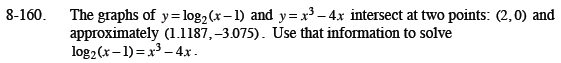Home > CCA2 > Chapter 8 > Lesson 8.3.2 > Problem8-160

8-160.

The graphs of y = log2(x − 1) and y = x3 − 4x intersect at two points: (2, 0) and approximately (1.1187, −3.075). Use that information to solve log2(x − 1) = x3 − 4x. Homework Help ✎Look at the x-values of the points.